M340L 3rd Midterm, April 7, 2003

1. The following two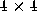matrices are row-equivalent: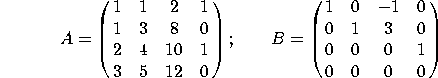a) What is the rank of A?

b) Find a basis for the column space of A.

c) Find a basis for the null space of A.

d) Find a basis for the row space of A.

2. Let V=Span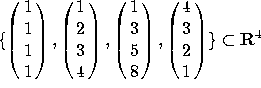.

a) What is the dimension of V?

b) Find a basis for V.

c) Let W be the subspace of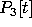spanned by the polynomials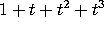,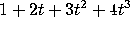,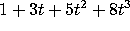and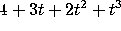. What is the dimension of W? Find a basis for W.

3. Consider the following basis for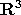: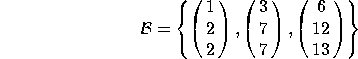a) Find the change-of-basis matrix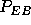that converts from coordinates in the B basis to coordinates in the standard (E) basis.

b) Fina the change-of-basis matrix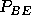that converts from coordinates in the standard basis to coordinates in the B basis.

c) Let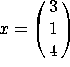. Compute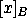.

d) Consider the basis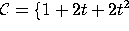,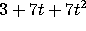,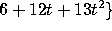for the vector space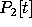. Find the coordinates of the polynomial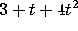in this basis.

4. a) Are the polynomials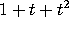,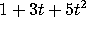and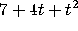linearly independent?

b) Do the polynomials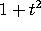,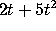and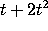span?

5. Indicate whether each of these statements is true or false. If a statement is sometimes true and sometimes false, write ``false''. You do NOT have to justify your answers. There is no penalty for wrong answers, so go ahead and guess if you are unsure of your answer.

a) The rank of a matrix is the dimension of its row space.

b) Let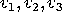be vectors in a vector space V. For Span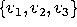to be a subspace of V, the vectors must be linearly independent.

c) If A and B are row-equivalent matrices, then Col(A)=Col(B).

d) If A and B are row-equivalent matrices, then Null(A)=Null(B).

e) If A and B are row-equivalent matrices, then Row(A)=Row(B).

f) Let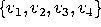be a collection of four vectors in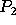. One of the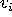's can be written as a linear combination of the others.

g) If a collection of five polynomials spans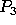, then it forms a basis for.

h) Every change-of-basis matrix is invertible.

i) If a linearly dependent set of vectors spans, then there must be at least 4 vectors in the set.

j) If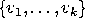is a linearly independent set of vectors in V, and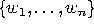is a spanning set for V, then n>k.